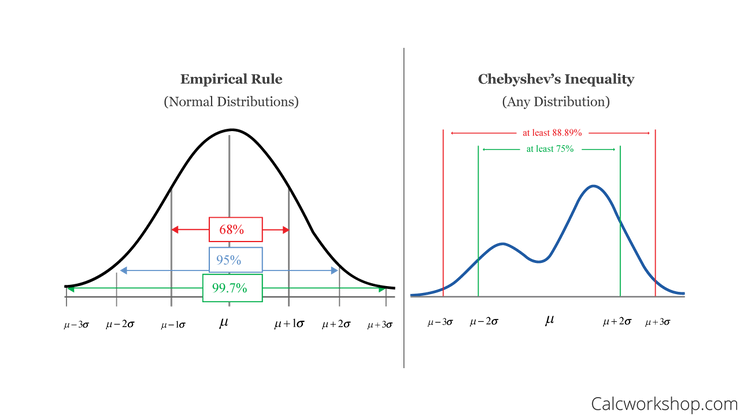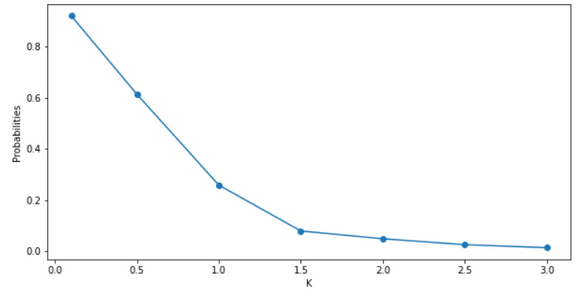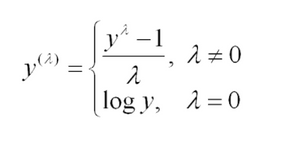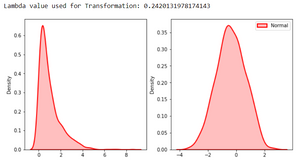# 6 Advanced Statistical Concepts in Data Science

The article contains some of the most commonly used advanced statistical concepts along with their Python implementation.

In my previous articles Beginners Guide to Statistics in Data Science and The Inferential Statistics Data Scientists Should Know we have talked about almost all the basics(Descriptive and Inferential) of statistics which are commonly used in understanding and working with any data science case study. In this article, lets go a little beyond and talk about some advance concepts which are not part of the buzz.

# Concept #1 -Q-Q(quantile-quantile) Plots

Before understanding QQ plots first understand what is a Quantile?

A quantile defines a particular part of a data set, i.e. a quantile determines how many values in a distribution are above or below a certain limit. Special quantiles are the quartile (quarter), the quintile (fifth), and percentiles (hundredth).

An example:

If we divide a distribution into four equal portions, we will speak of four quartiles. The first quartile includes all values that are smaller than a quarter of all values. In a graphical representation, it corresponds to 25% of the total area of distribution. The two lower quartiles comprise 50% of all distribution values. The interquartile range between the first and third quartile equals the range in which 50% of all values lie that are distributed around the mean.

In Statistics, A Q-Q(quantile-quantile) plot is a scatterplot created by plotting two sets of quantiles against one another. If both sets of quantiles came from the same distribution, we should see the points forming a line that’s roughly straight(y=x).For example, the median is a quantile where 50% of the data fall below that point and 50% lie above it. The purpose of Q Q plots is to find out if two sets of data come from the same distribution. A 45-degree angle is plotted on the Q Q plot; if the two data sets come from a common distribution, the points will fall on that reference line.

It’s very important for you to know whether the distribution is normal or not so as to apply various statistical measures on the data and interpret it in much more human-understandable visualization and their Q-Q plot comes into the picture. The most fundamental question answered by the Q-Q plot is if the curve is Normally Distributed or not.

Normally distributed, but why?

The Q-Q plots are used to find the type of distribution for a random variable whether it is a Gaussian Distribution, Uniform Distribution, Exponential Distribution, or even Pareto Distribution, etc.

You can tell the type of distribution using the power of the Q-Q plot just by looking at the plot. In general, we are talking about Normal distributions only because we have a very beautiful concept of the 68–95–99.7 rule which perfectly fits into the normal distribution So we know how much of the data lies in the range of the first standard deviation, second standard deviation and third standard deviation from the mean. So knowing if a distribution is Normal opens up new doors for us to experiment withTypes of Q-Q plots. Source

Skewed Q-Q plots

Q-Q plots can find skewness(measure of asymmetry) of the distribution.

If the bottom end of the Q-Q plot deviates from the straight line but the upper end is not, then the distribution is Left skewed(Negatively skewed).

Now if upper end of the Q-Q plot deviates from the staright line and the lower is not, then the distribution is Right skewed(Positively skewed).

Tailed Q-Q plots

Q-Q plots can find Kurtosis(measure of tailedness) of the distribution.

The distribution with the fat tail will have both the ends of the Q-Q plot to deviate from the straight line and its centre follows the line, where as a thin tailed distribution will term Q-Q plot with very less or negligible deviation at the ends thus making it a perfect fit for normal distribution.

Q-Q plots in Python(Source)

Suppose we have the following dataset of 100 values:

```import numpy as np

#create dataset with 100 values that follow a normal distribution
np.random.seed(0)
data = np.random.normal(0,1, 1000)

#view first 10 values
data[:10] ```

```array([ 1.76405235,  0.40015721,  0.97873798,  2.2408932 ,  1.86755799,
-0.97727788,  0.95008842, -0.15135721, -0.10321885,  0.4105985 ])```

To create a Q-Q plot for this dataset, we can use the qqplot() function from the statsmodels library:

```import statsmodels.api as sm
import matplotlib.pyplot as plt

#create Q-Q plot with 45-degree line added to plot
fig = sm.qqplot(data, line='45')
plt.show()```In a Q-Q plot, the x-axis displays the theoretical quantiles. This means it doesn’t show your actual data, but instead, it represents where your data would be if it were normally distributed.

The y-axis displays your actual data. This means that if the data values fall along a roughly straight line at a 45-degree angle, then the data is normally distributed.

We can see in our Q-Q plot above that the data values tend to closely follow the 45-degree, which means the data is likely normally distributed. This shouldn’t be surprising since we generated the 100 data values by using the numpy.random.normal() function.

Consider instead if we generated a dataset of 100 uniformly distributed values and created a Q-Q plot for that dataset:

```#create dataset of 100 uniformally distributed values
data = np.random.uniform(0,1, 1000)

#generate Q-Q plot for the dataset
fig = sm.qqplot(data, line='45')
plt.show()```The data values clearly do not follow the red 45-degree line, which is an indication that they do not follow a normal distribution.

# Concept #2- Chebyshev's Inequality

In probability, Chebyshev’s Inequality, also known as “Bienayme-Chebyshev” Inequality guarantees that, for a wide class of probability distributions, only a definite fraction of values will be found within a specific distance from the mean of a distribution.Chebyshev’s inequality is similar to The Empirical rule(68-95-99.7); however, the latter rule only applies to normal distributions. Chebyshev’s inequality is broader; it can be applied to any distribution so long as the distribution includes a defined variance and mean.

So Chebyshev’s inequality says that at least (1-1/k^2) of data from a sample must fall within K standard deviations from the mean (or equivalently, no more than 1/k^2 of the distribution’s values can be more than k standard deviations away from the mean).

Where K --> Positive real number

If the data is not normally distributed then different amounts of data could be in one standard deviation. Chebyshev’s inequality provides a way to know what fraction of data falls within K standard deviations from the mean for any data distribution.

Also read: 22 Statistics Questions to Prepare for Data Science InterviewsChebyshev’s inequality is of great value because it can be applied to any probability distribution in which the mean and variance are provided.

Let us consider an example, Assume 1,000 contestants show up for a job interview, but there are only 70 positions available. In order to select the finest 70 contestants amongst the total contestants, the proprietor gives tests to judge their potential. The mean score on the test is 60, with a standard deviation of 6. If an applicant scores an 84, can they presume that they are getting the job?The results show that about 63 people scored above a 60, so with 70 positions available, a contestant who scores an 84 can be assured they got the job.

Chebyshev's Inequality in Python(Source)

Create a population of 1,000,000 values, I use a gamma distribution(also works with other distributions) with shape = 2 and scale = 2.

```import numpy as np
import random
import matplotlib.pyplot as plt

#create a population with a gamma distribution
shape, scale = 2., 2.  #mean=4, std=2*sqrt(2)
mu = shape*scale #mean and standard deviation
sigma = scale*np.sqrt(shape)

s = np.random.gamma(shape, scale, 1000000)```

Now sample 10,000 values from the population.

```#sample 10000 values
rs = random.choices(s, k=10000)```

Count the sample that has a distance from the expected value larger than k standard deviation and use the count to calculate the probabilities. I want to depict a trend of probabilities when k is increasing, so I use a range of k from 0.1 to 3.

```#set k
ks = [0.1,0.5,1.0,1.5,2.0,2.5,3.0]

#probability list
probs = [] #for each k

for k in ks:
#start count
c = 0
for i in rs:
# count if far from mean in k standard deviation
if abs(i - mu) > k * sigma :
c += 1
probs.append(c/10000)```

Plot the results:

```plot = plt.figure(figsize=(20,10))
#plot each probability
plt.xlabel('K')
plt.ylabel('probability')
plt.plot(ks,probs, marker='o')
plot.show()
#print each probability
print("Probability of a sample far from mean more than k standard deviation:")
for i, prob in enumerate(probs):
print("k:" + str(ks[i]) + ", probability: " \
+ str(prob)[0:5] + \
" | in theory, probability should less than: " \
+ str(1/ks[i]**2)[0:5])```From the above plot and result, we can see that as the k increases, the probability is decreasing, and the probability of each k follows the inequality. Moreover, only the case that k is larger than 1 is useful. If k is less than 1, the right side of the inequality is larger than 1 which is not useful because the probability cannot be larger than 1.

# Concept #3-Log-Normal Distribution

In probability theory, a Log-normal distribution also known as Galton's distribution is a continuous probability distribution of a random variable whose logarithm is normally distributed.

Thus, if the random variable X is log-normally distributed, then Y = ln(X) has a normal distribution. Equivalently, if Y has a normal distribution, then the exponential function of Y i.e, X = exp(Y), has a log-normal distribution.

Skewed distributions with low mean and high variance and all positive values fit under this type of distribution. A random variable that is log-normally distributed takes only positive real values.

The general formula for the probability density function of the lognormal distribution is:The location and scale parameters are equivalent to the mean and standard deviation of the logarithm of the random variable.

The shape of Lognormal distribution is defined by 3 parameters:

1. σis the shape parameter, (and is the standard deviation of the log of the distribution)

2. θ or μ is the location parameter (and is the mean of the distribution)

3. m is the scale parameter (and is also the median of the distribution)

The location and scale parameters are equivalent to the mean and standard deviation of the logarithm of the random variable as explained above.

If x = θ, then f(x) = 0. The case where θ = 0 and m = 1 is called the standard lognormal distribution. The case where θ equals zero is called the 2-parameter lognormal distribution.

The following graph illustrates the effect of the location(μ) and scale(σ) parameter on the probability density function of the lognormal distribution:Log-Normal Distribution in Python(Source)

Let us consider an example to generate random numbers from a log-normal distribution with μ=1 and σ=0.5 using scipy.stats.lognorm function.

```import numpy as np
import matplotlib.pyplot as plt
from scipy.stats import lognorm

np.random.seed(42)

data = lognorm.rvs(s=0.5, loc=1, scale=1000, size=1000)

plt.figure(figsize=(10,6))
ax = plt.subplot(111)
plt.title('Generate wrandom numbers from a Log-normal distribution')
ax.hist(data, bins=np.logspace(0,5,200), density=True)
ax.set_xscale("log")

shape,loc,scale = lognorm.fit(data)

x = np.logspace(0, 5, 200)
pdf = lognorm.pdf(x, shape, loc, scale)

ax.plot(x, pdf, 'y')
plt.show()```# Concept #4- Power Law distribution

In statistics, a Power Law is a functional relationship between two quantities, where a relative change in one quantity results in a proportional relative change in the other quantity, independent of the initial size of those quantities: one quantity varies as a power of another.

For instance, considering the area of a square in terms of the length of its side, if the length is doubled, the area is multiplied by a factor of four.

A power law distribution has the form Y = k Xα

where:

• X and Y are variables of interest,

• α is the law’s exponent,

• k is a constant.Power-law distribution is just one of many probability distributions, but it is considered a valuable tool to assess uncertainty issues that normal distribution cannot handle when they occur at a certain probability.

Many processes have been found to follow power laws over substantial ranges of values. From the distribution in incomes, size of meteoroids, earthquake magnitudes, the spectral density of weight matrices in deep neural networks, word usage, number of neighbors in various networks, etc. (Note: The power law here is a continuous distribution. The last two examples are discrete, but on a large scale can be modeled as if continuous).

Also read: Statistical Measures of Central Tendency

Power-law distribution in Python(Source)

Let us plot the Pareto distribution which is one form of a power-law probability distribution. Pareto distribution is sometimes known as the Pareto Principle or ‘80–20’ rule, as the rule states that 80% of society’s wealth is held by 20% of its population. Pareto distribution is not a law of nature, but an observation. It is useful in many real-world problems. It is a skewed heavily tailed distribution.

```import numpy as np
import matplotlib.pyplot as plt
from scipy.stats import pareto

x_m = 1 #scale
alpha = [1, 2, 3] #list of values of shape parameters
plt.figure(figsize=(10,6))
samples = np.linspace(start=0, stop=5, num=1000)
for a in alpha:
output = np.array([pareto.pdf(x=samples, b=a, loc=0, scale=x_m)])
plt.plot(samples, output.T, label='alpha {0}' .format(a))

plt.xlabel('samples', fontsize=15)
plt.ylabel('PDF', fontsize=15)
plt.title('Probability Density function', fontsize=15)
plt.legend(loc='best')
plt.show()```# Concept #5- Box cox transformation

The Box-Cox transformation transforms our data so that it closely resembles a normal distribution.

The one-parameter Box-Cox transformations are defined as In many statistical techniques, we assume that the errors are normally distributed. This assumption allows us to construct confidence intervals and conduct hypothesis tests. By transforming your target variable, we can (hopefully) normalize our errors (if they are not already normal).

Additionally, transforming our variables can improve the predictive power of our models because transformations can cut away white noise.Original distribution(Left) and near-normal distribution after applying Box cox transformation. Source

At the core of the Box-Cox transformation is an exponent, lambda (λ), which varies from -5 to 5. All values of λ are considered and the optimal value for your data is selected; The “optimal value” is the one that results in the best approximation of a normal distribution curve.

The one-parameter Box-Cox transformations are defined as:and the two-parameter Box-Cox transformations as:Moreover, the one-parameter Box-Cox transformation holds for y > 0, i.e. only for positive values and two-parameter Box-Cox transformation for y > -λ, i.e. negative values.

The parameter λ is estimated using the profile likelihood function and using goodness-of-fit tests.

If we talk about some drawbacks of Box-cox transformation, then if interpretation is what you want to do, then Box-cox is not recommended. Because if λ is some non-zero number, then the transformed target variable may be more difficult to interpret than if we simply applied a log transform.

A second stumbling block is that the Box-Cox transformation usually gives the median of the forecast distribution when we revert the transformed data to its original scale. Occasionally, we want the mean and not the median.

Box-Cox transformation in Python(Source)

SciPy’s stats package provides a function called boxcox for performing box-cox power transformation that takes in original non-normal data as input and returns fitted data along with the lambda value that was used to fit the non-normal distribution to normal distribution.

```#load necessary packages
import numpy as np
from scipy.stats import boxcox
import seaborn as sns

#make this example reproducible
np.random.seed(0)

#generate dataset
data = np.random.exponential(size=1000)

fig, ax = plt.subplots(1, 2)
#plot the distribution of data values
sns.distplot(data, hist=False, kde=True,
kde_kws = {'shade': True, 'linewidth': 2},
label = "Non-Normal", color ="red", ax = ax)

#perform Box-Cox transformation on original data
transformed_data, best_lambda = boxcox(data)

sns.distplot(transformed_data, hist = False, kde = True,
kde_kws = {'shade': True, 'linewidth': 2},
label = "Normal", color ="red", ax = ax)

#adding legends to the subplots
plt.legend(loc = "upper right")

#rescaling the subplots
fig.set_figheight(5)
fig.set_figwidth(10)
#display optimal lambda value
print(f"Lambda value used for Transformation: {best_lambda}")
```# Concept #6- Poisson distribution

In probability theory and statistics, the Poisson distribution is a discrete probability distribution that expresses the probability of a given number of events occurring in a fixed interval of time or space if these events occur with a known constant mean rate and independently of the time since the last event.

In very simple terms, A Poisson distribution can be used to estimate how likely it is that something will happen "X" number of times.

Some examples of Poisson processes are customers calling a help center, radioactive decay in atoms, visitors to a website, photons arriving at a space telescope, and movements in a stock price. Poisson processes are usually associated with time, but they do not have to be.

The Formula for the Poisson Distribution Is:Where:

• e is Euler's number (e = 2.71828...)

• k is the number of occurrences

• k! is the factorial of k

• λ is equal to the expected value of kwhen that is also equal to its variance

Lambda(λ) can be thought of as the expected number of events in the interval. As we change the rate parameter, λ, we change the probability of seeing different numbers of events in one interval. The below graph is the probability mass function of the Poisson distribution showing the probability of a number of events occurring in an interval with different rate parameters.Probability Mass function for Poisson Distribution with varying rate parameters.Source

The Poisson distribution is also commonly used to model financial count data where the tally is small and is often zero. For one example, in finance, it can be used to model the number of trades that a typical investor will make in a given day, which can be 0 (often), or 1, or 2, etc.

As another example, this model can be used to predict the number of "shocks" to the market that will occur in a given time period, say over a decade.

Poisson distribution in Python

```from numpy import random
import matplotlib.pyplot as plt
import seaborn as sns

lam_list = [1, 4, 9] #list of Lambda values

plt.figure(figsize=(10,6))
samples = np.linspace(start=0, stop=5, num=1000)

for lam in lam_list:
sns.distplot(random.poisson(lam=lam, size=10), hist=False, label='lambda {0}'.format(lam))

plt.xlabel('Poisson Distribution', fontsize=15)
plt.ylabel('Frequency', fontsize=15)
plt.legend(loc='best')
plt.show()```As λ becomes bigger, the graph looks more like a normal distribution.

Also read: False Positives vs. False Negatives

Feel free to connect me on LinkedIn for any query.

References

“6 Advanced Statistical Concepts in Data Science”
– Nagesh Singh Chauhan

1.Daniel Morales
11 months ago
Hi medouard, you're welcome!
2.medouard
11 months ago
Thanks a lot for this article, it's usefull. :)

### Related Stories

Nov 25, 2021

#### 5 Tips To Ace Your Job Interview For A Data Scientist Opening

5 Tips To Ace Your Job Interview For A Data Scientist Opening.PNG 795.94 KBImage SourceAspiring data scientists have a bright future ahead of them....By Daniel Morales
Nov 12, 2021

#### When to Avoid Deep Learning

IntroductionThis article is intended for data scientists who may consider using deep learning algorithms, and want to know more about the cons of i...By Matt Przybyla
Oct 09, 2021

#### Top 10 Python Extensions for Visual Studio Code

In this new post we want to talk about the most useful Python extensions for Visual Studio Code. Visual Studio Code is an integrated development en...By Daniel Morales### Join our private community in Slack

#### Keep up to date by participating in our global community of data scientists and AI enthusiasts. We discuss the latest developments in data science competitions, new techniques for solving complex challenges, AI and machine learning models, and much more!

We'll send you an invitational link to your email immediatly.